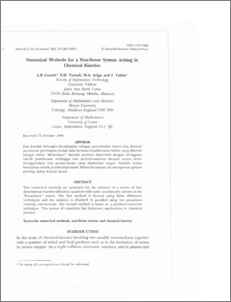# Numerical Methods for a Non-linear System Arising in Chemical Kinetics

## Citation

Gumel, A. B. and Twizell, E. H. and Arigu, M. A. and Fakhir, F. (1997) Numerical Methods for a Non-linear System Arising in Chemical Kinetics. Pertanika Journal of Science & Technology, 5 (2). pp. 191-200. ISSN 0128-7680

## Abstract

Two numerical methods are proposed for the solution of a system of two dimensional reaction-diffusion equations with cubic non-linearity, known as the "Brusselator" system. The first method is derived using finite difference techniques and the solution is obtained in paralled using two processors running concurrently. The second method is based on a predictor-eorrector technique. This system of equations has important applications in chemical kinetics.Preview
PDF
Numerical_Methods_for_a_Non-linear_System_Arising_in.pdfView Item